0
0
01. 云栖社区>
2. 博客>
3. 正文

超级课程表APP爬虫，大学生都这么玩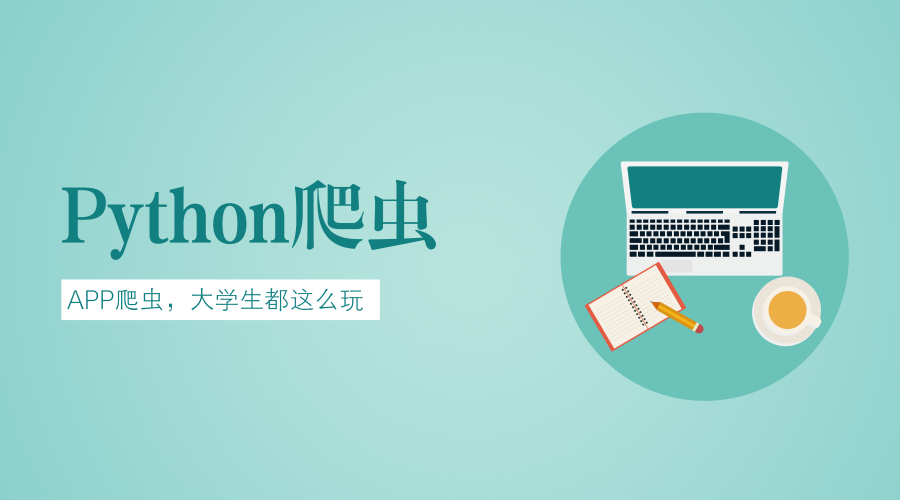• 爬虫简介
• 性别分布
• 高校分布
• 帖子时间
• 帖子词云

爬虫简介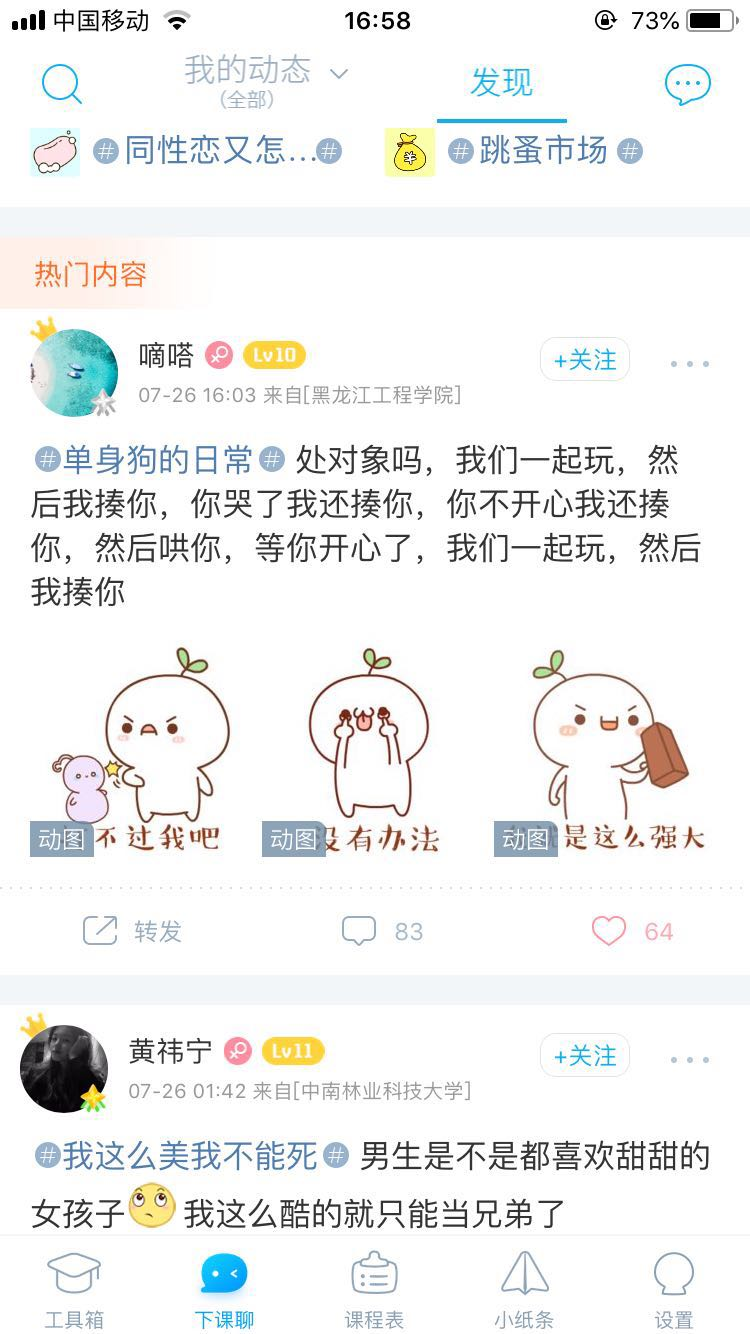数据分析

• 用户名
• 性别（0为女性）
• 学校
• 发帖时间
• 发帖内容
• 发帖标签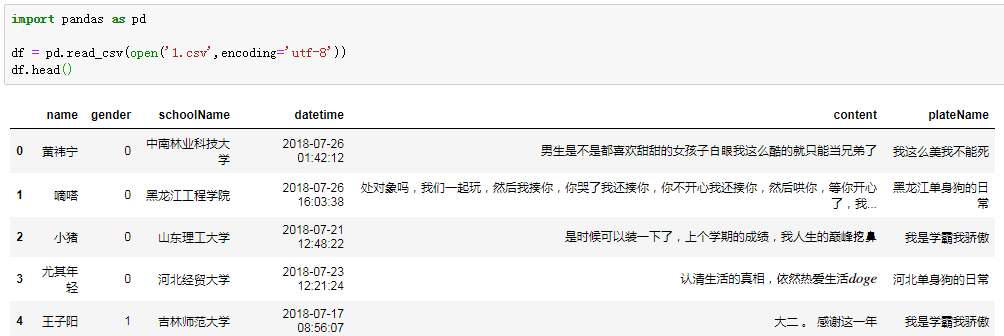性别分布

data1 = df.groupby('gender')['gender'].count()

from pyecharts import Pie

attr = ['女生', '男生']
v1 = list(data1)
pie = Pie('用户性别分布')
pie.add("", attr, v1, is_label_show=True)
pie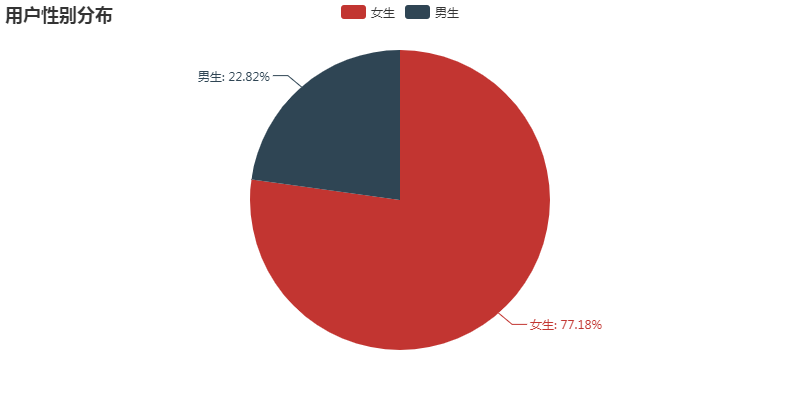高校分布

data2 = df.groupby('schoolName')['schoolName'].count()
data2 = data2.sort_values(ascending=False)[:10]

from pyecharts import Bar

bar = Bar('所在学校排行')
attr = list(data2.index)
v2 = list(data2)
bar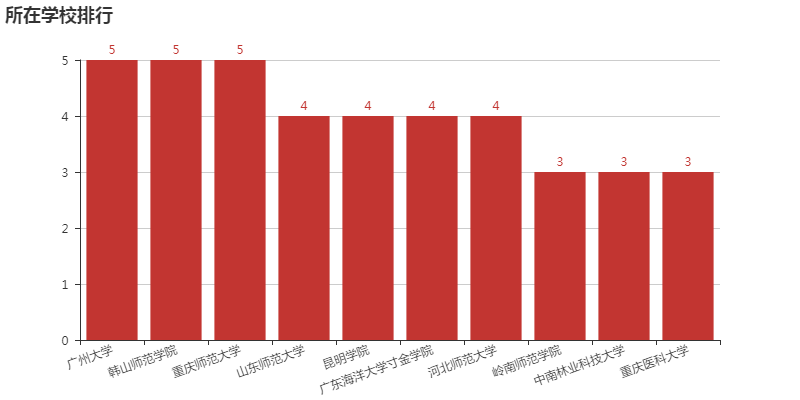帖子时间

df['hour'] = df['datetime'].str.split(':').str.str.split(' ').str
data3 = df['hour'].value_counts()
data3 = data3.sort_index()
from pyecharts import Line

attr = list(data3.index)
v = list(data3)

line = Line("帖子发布时间分布")
line帖子词云

• 学习备考考研约图书馆
• 单身小哥哥小姐姐求脱单
如果你要问我doge是什么，可能就是屏幕前的你吧。from pyecharts import WordCloud

wordcloud = WordCloud(width=800, height=620)
wordcloud.add("", label, attr, word_size_range=[20, 100])
wordcloud讨论

• 数据不多，代表性可能不强
• 珍惜大学时光，不要做后悔之事

+ 关注

corcosa 10037人浏览